# Example: Completing perfect square trinomials

##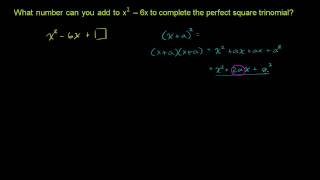By Khan Academy

This is a Khan Academy video on completing perfect square trinomials.# Solving quadratics by completing the square 1

##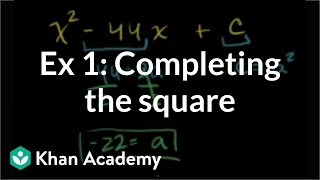By Khan Academy

Sal uses completing the square to find the value of c that makes x^2-44x+c a perfect square.# Math 8 Honors: Chapter 9.8- Completing the Square PART 1

##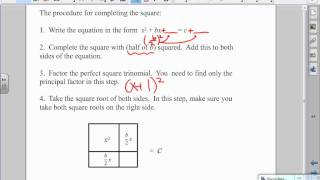By Megan Frantz

Completing the Square# Math 8 Honors: Chapter 9.8- Completing the Square PART 2

##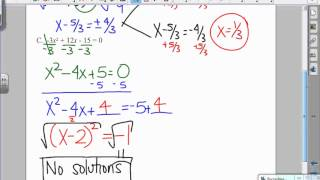By Megan Frantz

Completing the Square# Ex 3: Completing the Square - Complex Solutions

##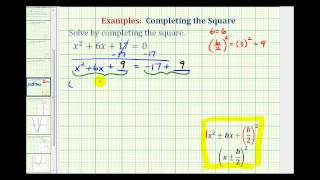By Mathispower4u

Ex 3: Completing the Square - Complex Solutions# Completing The Square - YourTeacher.com - Algebra Help

##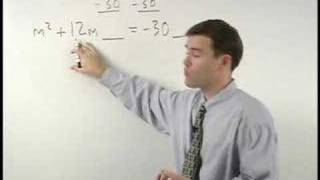By yourteachermathhelp

Youtube Presents Completing The Square - YourTeacher.com - Algebra Help an educational video resources on math.# Completing The Square - YourTeacher.com - Algebra Help

##By yourteachermathhelp

Youtube Presents Completing The Square - YourTeacher.com - Algebra Help an educational video resources on math.# Solving quadratics by completing the square 2

##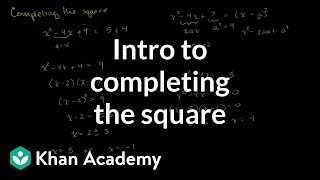By Khan Academy

Sal explains what's completing the square of a quadratic expression, how to do it, and why it's so helpful!# Completing The Square - YourTeacher.com - Algebra Help

##By yourteachermathhelp

Youtube Presents Completing The Square - YourTeacher.com - Algebra Help an educational video resources on math.# Example 1: Completing the square | Quadratic equations | Algebra I | Khan Academy

##By Khan Academy

Completing the Square 2-From Khan Academy# Completing the square for quadratic formula | Quadratic equations | Algebra I | Khan Academy

##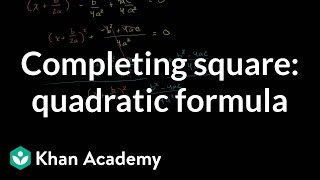By Khan Academy

Completing the Square 4-From Khan Academy# Completing the square for quadratic formula | Quadratic equations | Algebra I | Khan Academy

##By Khan Academy

Completing the Square 4-From Khan Academy# Completing the square for vertex form | Quadratic equations | Algebra I | Khan Academy

##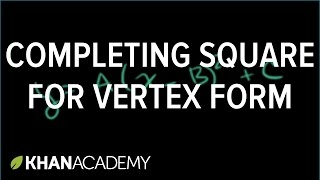By Khan Academy

An example problem on how to complete the square to write a quadratic function in vertex form.# Completing the square for vertex form | Quadratic equations | Algebra I | Khan Academy

##By Khan Academy

An example problem on how to complete the square to write a quadratic function in vertex form.# Finding the Center-Radius Form of a Circle by Completing the Square - Example 1

##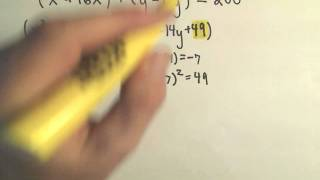By PatrickJMT

YouTube presents Finding the Center-Radius Form of a Circle by Completing the Square - Example 1, an educational video resource on math.# Example 2: Completing the square | Quadratic equations | Algebra I | Khan Academy

##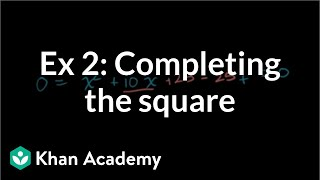By Khan Academy

This Khan Academy video offers more information on completing the square and contains a sample problem with a walkthrough.# Example 2: Completing the square | Quadratic equations | Algebra I | Khan Academy

##By Khan Academy

This Khan Academy video offers more information on completing the square and contains a sample problem with a walkthrough.# Example 3: Completing the square | Quadratic equations | Algebra I | Khan Academy

##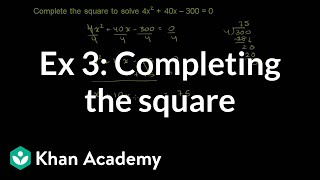By Khan Academy

The following resource is a video from Khan Academy that shows how to complete the square to solve quadratic equations.# Finding the Center-Radius Form of a Circle by Completing the Square - Example 3

##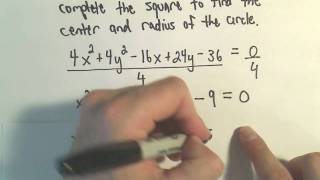By PatrickJMT

Youtube Presents Finding the Center-Radius Form of a Circle by Completing the Square - Example 3 an educational video resources on english language arts.# Finding the Center-Radius Form of a Circle by Completing the Square - Example 2

##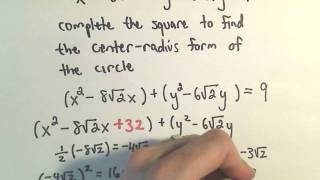By PatrickJMT

Youtube Presents Finding the Center-Radius Form of a Circle by Completing the Square - Example 2 an educational video resources on english language arts.